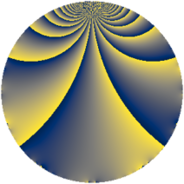# Properties

 Label 930.2.oLevel $930$ Weight $2$ Character orbit 930.o Rep. character $\chi_{930}(161,\cdot)$ Character field $\Q(\zeta_{6})$ Dimension $88$ Newform subspaces $5$ Sturm bound $384$ Trace bound $6$

# Related objects

## Defining parameters

 Level: $$N$$ $$=$$ $$930 = 2 \cdot 3 \cdot 5 \cdot 31$$ Weight: $$k$$ $$=$$ $$2$$ Character orbit: $$[\chi]$$ $$=$$ 930.o (of order $$6$$ and degree $$2$$) Character conductor: $$\operatorname{cond}(\chi)$$ $$=$$ $$93$$ Character field: $$\Q(\zeta_{6})$$ Newform subspaces: $$5$$ Sturm bound: $$384$$ Trace bound: $$6$$ Distinguishing $$T_p$$: $$7$$, $$11$$

## Dimensions

The following table gives the dimensions of various subspaces of $$M_{2}(930, [\chi])$$.

Total New Old
Modular forms 400 88 312
Cusp forms 368 88 280
Eisenstein series 32 0 32

## Trace form

 $$88q - 88q^{4} - 8q^{7} + O(q^{10})$$ $$88q - 88q^{4} - 8q^{7} + 88q^{16} - 16q^{18} - 16q^{19} + 60q^{21} + 44q^{25} + 8q^{28} + 24q^{31} - 8q^{33} + 12q^{34} - 16q^{39} + 24q^{42} - 8q^{45} - 60q^{49} + 16q^{51} + 12q^{55} - 12q^{57} + 112q^{63} - 88q^{64} - 32q^{66} + 56q^{67} - 4q^{69} + 16q^{72} + 120q^{73} + 16q^{76} - 48q^{78} - 12q^{79} - 48q^{81} - 8q^{82} - 60q^{84} + 8q^{87} - 8q^{90} + 32q^{93} - 24q^{94} - 96q^{97} - 108q^{99} + O(q^{100})$$

## Decomposition of $$S_{2}^{\mathrm{new}}(930, [\chi])$$ into newform subspaces

Label Dim. $$A$$ Field CM Traces $q$-expansion
$$a_2$$ $$a_3$$ $$a_5$$ $$a_7$$
930.2.o.a $$4$$ $$7.426$$ $$\Q(\zeta_{12})$$ None $$0$$ $$-6$$ $$0$$ $$8$$ $$q+\zeta_{12}^{3}q^{2}+(-1-\zeta_{12}^{2})q^{3}-q^{4}+\cdots$$
930.2.o.b $$4$$ $$7.426$$ $$\Q(\zeta_{12})$$ None $$0$$ $$0$$ $$0$$ $$2$$ $$q+\zeta_{12}^{3}q^{2}+(-\zeta_{12}+2\zeta_{12}^{3})q^{3}+\cdots$$
930.2.o.c $$4$$ $$7.426$$ $$\Q(\zeta_{12})$$ None $$0$$ $$0$$ $$0$$ $$2$$ $$q+\zeta_{12}^{3}q^{2}+(\zeta_{12}-2\zeta_{12}^{3})q^{3}-q^{4}+\cdots$$
930.2.o.d $$36$$ $$7.426$$ None $$0$$ $$0$$ $$0$$ $$-8$$
930.2.o.e $$40$$ $$7.426$$ None $$0$$ $$6$$ $$0$$ $$-12$$

## Decomposition of $$S_{2}^{\mathrm{old}}(930, [\chi])$$ into lower level spaces

$$S_{2}^{\mathrm{old}}(930, [\chi]) \cong$$ $$S_{2}^{\mathrm{new}}(93, [\chi])$$$$^{\oplus 4}$$$$\oplus$$$$S_{2}^{\mathrm{new}}(186, [\chi])$$$$^{\oplus 2}$$$$\oplus$$$$S_{2}^{\mathrm{new}}(465, [\chi])$$$$^{\oplus 2}$$